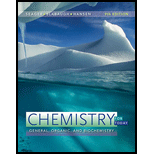# The oxidation number of sulfur in the ion SO 4 − 2 is: a. − 2 b. + 2 c. + 6 d. + 10### Chemistry for Today: General, Orga...

9th Edition
Spencer L. Seager + 2 others
Publisher: Cengage Learning
ISBN: 9781305960060

#### Solutions

Chapter
Section### Chemistry for Today: General, Orga...

9th Edition
Spencer L. Seager + 2 others
Publisher: Cengage Learning
ISBN: 9781305960060
Chapter 5, Problem 5.80E
Textbook Problem
1 views

## The oxidation number of sulfur in the ion SO 4 − 2 is:a. − 2 b. + 2 c. + 6 d. + 10

Interpretation Introduction

Interpretation:

The option corresponding to the correct oxidation number of sulfur in the ion SO42 is to be identified.

Concept Introduction:

The oxidation state or number in a compound is the charge present on the individual atom if all the bonds are assumed to be ionic. The oxidation number is assigned by the following set of rules:

1. The oxidation number present on a neutral compound is always zero.

2. The oxidation number of atoms present in an element in the pure form is zero.

3. In the compounds, if halogens are present the oxidation number of halogens is 1, but when oxygen is present with them they have different oxidation number.

4. The oxidation numbers of Group 1 metals are always +1 and Group 2 metals are always +2.

5. The common oxidation number of oxygen in most compounds is 2 and oxidation number of hydrogen is +1.

### Explanation of Solution

The oxidation number of sulfur in the ion SO42 is calculated by the formula,

(Sum of oxidation number of allelements present in acompound)=Net charge on compound

In SO42, the oxidation number of sulfur is assumed to be x.

The oxidation number of oxygen is 2.

Substitute the values in the above formula,

### Still sussing out bartleby?

Check out a sample textbook solution.

See a sample solution

#### The Solution to Your Study Problems

Bartleby provides explanations to thousands of textbook problems written by our experts, many with advanced degrees!

Get Started

Find more solutions based on key concepts
Irradiation can a. destroy vitamins. b. sterilize spices. c. make food radioactive. d. promote sprouting.

Nutrition: Concepts and Controversies - Standalone book (MindTap Course List)

How many autosomes are present in a body cell of a human being? In a gamete?

Human Heredity: Principles and Issues (MindTap Course List)

Review. A light, unstressed spring has length d. Two identical particles, each with charge q, are connected to ...

Physics for Scientists and Engineers, Technology Update (No access codes included)